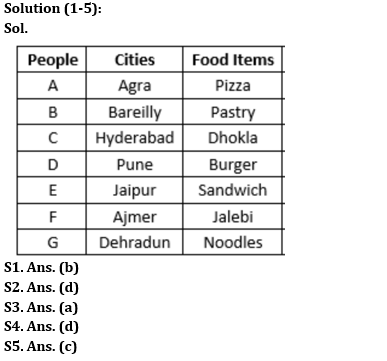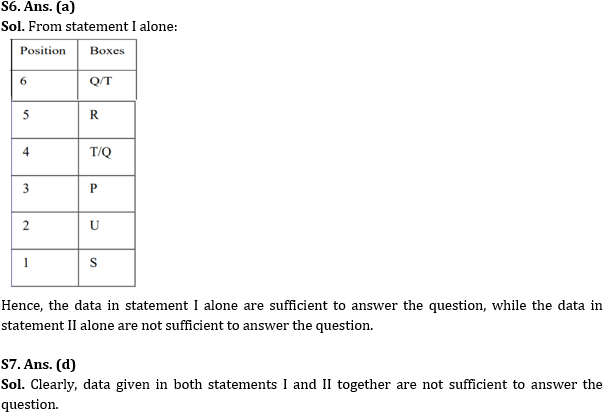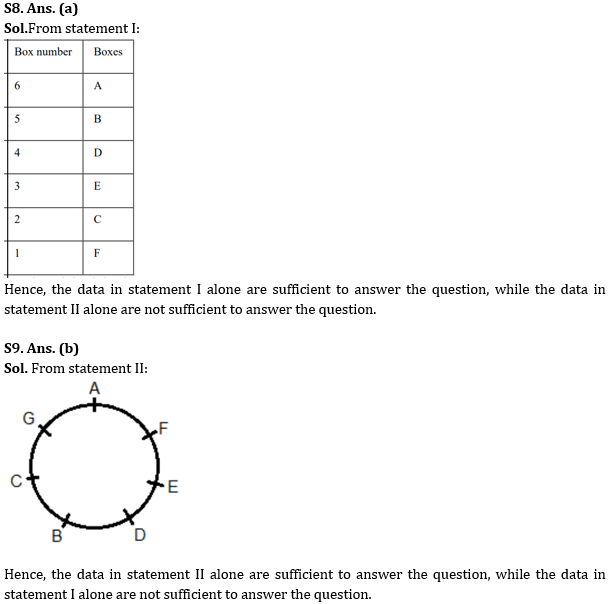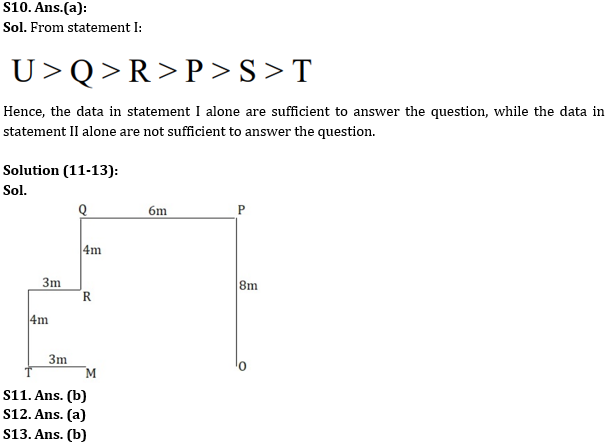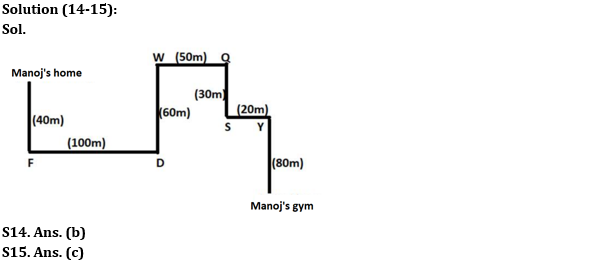Latest Banking jobs   »   Reasoning Ability Quiz For RBI Grade...

# Reasoning Ability Quiz For RBI Grade B/ ECGC PO/ SIDBI Grade A Prelims 2022- 2nd May

Directions (1-5): Study the following information carefully and answer the questions given below:
Seven people A, B, C, D, E, F and G belong to different cities i.e., Pune, Hyderabad, Jaipur, Ajmer, Dehradun, Agra and Bareilly. They all like different food items i.e., Jalebi, Dhokla, Pastry, Pizza, Burger, Sandwich and Noodles. All the information is not necessarily in the same order. B likes Pastry but doesn’t belong to Agra. E belongs to Jaipur. C neither likes Jalebi nor Pizza. G belongs to Dehradun. D likes Burger. The one who belongs to Agra likes Pizza. F doesn’t like Pizza. The one who belongs to Ajmer likes Jalebi. The one who belongs to Hyderabad likes Dhokla. D doesn’t belong to Bareilly. G doesn’t like Sandwich.

Q1. Who amongst the following likes Noodles?
(a) E
(b) G
(c) B
(d) C
(e) None of these

Q2. Who amongst the following belongs to Pune?
(a) G
(b) B
(c) F
(d) D
(e) None of these

Q3. Which amongst the following pair is not correct?
(a) D – Dehradun – Burger
(b) G – Dehradun – Noodles
(c) B – Bareilly – Pastry
(d) All are correct
(e) None is correct

Q4. The one who likes Sandwich belongs to which amongst the following cities?
(b) Pune
(c) Bareilly
(d) Jaipur
(e) None of these

Q5. Four of the following five are alike in a certain way and hence form a group. Which is the one that doesn’t belong to that group?
(a) B – Agra
(c) E – Jaipur
(e) C – Pune

Directions (6-10): Each of the questions below consists of a question and two statements numbered I and II given below it. You have to decide whether the data provided in the statements are sufficient to answer the question. Read all the statements and answer the following questions.

Q6. Six boxes, P, Q, R, S, T and U are placed one above another where the bottommost position is 1 and the position just above it is 2 and so on but not necessarily in the same order. Which box is placed at the bottommost position?
I. R is placed 2nd to the above of P which is placed just above of U. One box is placed between Q and T. S is placed below T.
II. Two boxes are placed between Q and P. One box is placed between R and P.

(a) The data in statement I alone are sufficient to answer the question while the data in statement II alone are not sufficient to answer the question.
(b) The data in statement II alone are sufficient to answer the question while the data in statement I alone are not sufficient to answer the question.
(c) The data either in statement I alone or in statement II alone are sufficient to answer the question.
(d) The data given in both statements I and II together are not sufficient to answer the question.
(e) The data in both statements I and II together are necessary to answer the question.

Q7. Six persons, A, B, C, D, E and F are of different heights. Who is the 3rd shortest person?
I. A and C are shorter than D. F is not taller than E. B is taller than E but not the tallest.
II. B is taller than A and is the 2nd tallest person. F is shorter than D. C is not the tallest person. E is shorter than A and C. E is not the shortest.

(a) The data in statement I alone are sufficient to answer the question while the data in statement II alone are not sufficient to answer the question.
(b) The data in statement II alone are sufficient to answer the question while the data in statement I alone are not sufficient to answer the question.
(c) The data either in statement I alone or in statement II alone are sufficient to answer the question.
(d) The data given in both statements I and II together are not sufficient to answer the question.
(e) The data in both statements I and II together are necessary to answer the question.

Q8. Six boxes A, B, C, D, E and F are kept one above one another such that bottommost box is numbered as 1 while the topmost box is numbered as 6 but not necessarily in the same order. Which box is kept just below box C?
I. Box A is just above box B, which is an odd number box. Box D is three boxes above box F but not the topmost box. Box E is just above box C.
II. Box A is three boxes above box E. Box B is above box E. Box F is below box E.

(a) The data in statement I alone are sufficient to answer the question while the data in statement II alone are not sufficient to answer the question.
(b) The data in statement II alone are sufficient to answer the question while the data in statement I alone are not sufficient to answer the question.
(c) The data either in statement I alone or in statement II alone are sufficient to answer the question.
(d) The data given in both statements I and II together are not sufficient to answer the question.
(e) The data in both statements I and II together are necessary to answer the question.

Q9. Seven persons -A, B, C, D, E, F and G sit around a circular table facing towards the centre but not necessarily in the same order. Who sits immediate right of F?
I. G sits 2nd to the right of F. Two persons sit between C and E. D sits adjacent to E and to the immediate right of B.
II. B sits 4th to the right of F. G sits 2nd to the left of B. D sits to the immediate left of E. C doesn’t sit next to F.

(a) The data in statement I alone are sufficient to answer the question while the data in statement II alone are not sufficient to answer the question.
(b) The data in statement II alone are sufficient to answer the question while the data in statement I alone are not sufficient to answer the question.
(c) The data either in statement I alone or in statement II alone are sufficient to answer the question.
(d) The data given in both statements I and II together are not sufficient to answer the question.
(e) The data in both statements I and II together are necessary to answer the question.

Q10. Six persons P, Q, R, S, T and U have different marks in a class test. Who has 2nd highest marks?
I. R has more marks than S and T. At least two persons have more marks than R. P has 3rd lowest marks. Q doesn’t have the highest marks. S doesn’t have the lowest marks.
II. Three persons have lesser marks than R. Neither Q nor U has fewer marks than R. P has more marks than S and T.

(a) The data in statement I alone are sufficient to answer the question while the data in statement II alone are not sufficient to answer the question.
(b) The data in statement II alone are sufficient to answer the question while the data in statement I alone are not sufficient to answer the question.
(c) The data either in statement I alone or in statement II alone are sufficient to answer the question.
(d) The data given in both statements I and II together are not sufficient to answer the question.
(e) The data in both statements I and II together are necessary to answer the question.

Directions (11-13): Study the information given below and answer the questions based on it.
A person is standing at point P. He starts walking towards his west and walks for 6m and reached point Q and then he takes a left turn and walks for 4m and reached point R, then he takes a right turn and walks for 3m then he takes a left turn and walks for 4m and reached point T and then he takes another left turn and walks for 3m and reached point M.

Q11. Point O is in south direction of point P in a way that point T, M and O are in a horizontal line, then how far is point O with respect to point M?
(a) 4m
(b) 6m
(c) 5m
(d) 7m
(e) 11m

Q12. In which of the following direction is point M with respect to point P?
(a) South-west
(b) South-east
(c) North
(d) East
(e) North-west

Q13. In which of the following direction is point Q with respect to point T?
(a) North-west
(b) North-east
(c) South-east
(d) North
(e) South-west

Directions (14-15): Study the information given below and answer the questions based on it.
Manoj started walking from his home towards south and walked for 40m and reached Point F, then he turned towards his left and walked for 100m to reach Point D, after that he turned towards his left and walked for 60m to reach Point W, then he turned towards east and walked for 50m to reach Point Q, after that he turned towards his right for 30m and reached Point S and then he turned towards his left for 20m and reached Point Y finally took a right turn and walked for 80m to reach the gym.

Q14. What is the direction of Manoj’s gym with respect to the Manoj’s home?
(a) North-east
(b) South-east
(c) North-west
(d) South
(e) Cannot be determined

Q15. If point M is to the 20m west of the Manoj’s gym then what is the shortest distance between Point Q and Point M?
(a) 80m
(b) 100m
(c) 110m
(d) 120m
(e) None of these

Solutions